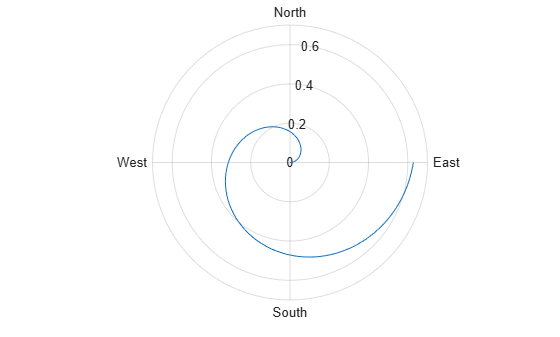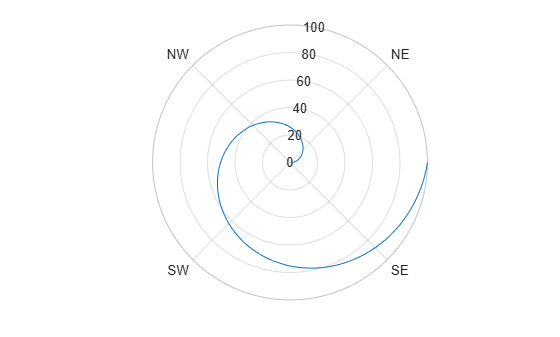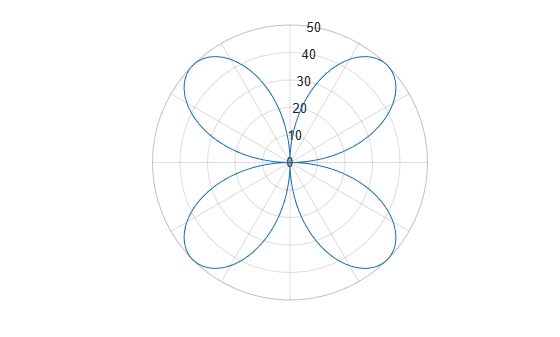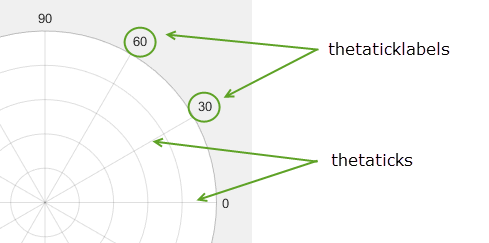# thetaticklabels

Set or query theta-axis tick labels

## Syntax

thetaticklabels(labels)
tl = thetaticklabels
thetaticklabels('auto')
thetaticklabels('manual')
m = thetaticklabels('mode')
___ = thetaticklabels(pax,___)

## Description

example

thetaticklabels(labels) sets the theta-axis tick labels for the current axes. Specify labels as a string array or a cell array of character vectors; for example, {'E','N','W','S'}. If you specify the labels, then the theta-axis tick values and tick labels no longer update automatically based on changes to the axes.
tl = thetaticklabels returns the theta-axis tick labels for the current axes.

example

thetaticklabels('auto') sets an automatic mode, enabling the axes to determine the theta-axis tick labels. Use this option if you set the labels and then want to set them back to the default values.
thetaticklabels('manual') sets a manual mode, freezing the theta-axis tick labels at the current values.
m = thetaticklabels('mode') returns the current value of the theta-axis tick labels mode, which is either 'auto' or 'manual'. By default, the mode is automatic unless you specify the tick labels or set the mode to manual.

example

___ = thetaticklabels(pax,___) uses the axes specified by pax instead of the current axes. Specify ax as the first input argument for any of the previous syntaxes.

## Examples

collapse all

Create a polar plot. Display tick marks and grid lines along the theta-axis at 0, 90, 180, and 270 degrees. Then, specify a label for each tick mark.

theta = linspace(0,2*pi); rho = theta/10; polarplot(theta,rho) thetaticks([0 90 180 270]) thetaticklabels({'East','North','West','South'})Create a polar plot and assign the polar axes object to the variable pax. Then, specify the theta-axis tick values and labels for the polar axes. Ensure that the thetaticks and thetaticklabels functions affect pax by passing the polar axes object as the first input argument to the functions.

theta = 0:0.01:2*pi; rho = 50*sin(2*theta); polarplot(theta,rho) pax = gca; thetaticks(pax,[0 90 180 270]) thetaticklabels(pax,{'East','North','West','South'})Create a polar plot and specify the theta-axis tick values and corresponding labels. Then, set the theta-axis tick values and labels back to the default values.

polarplot(1:100) thetaticks([45 135 225 315]) thetaticklabels({'NE','NW','SW','SE'})thetaticks('auto') thetaticklabels('auto')Remove the tick labels along the theta-axis by specifying the tick labels as an empty array.

theta = 0:0.01:2*pi; rho = 50*sin(2*theta); polarplot(theta,rho) thetaticklabels({})## Input Arguments

collapse all

Tick labels, specified as a cell array of character vectors, string array, or categorical array. If you do not want tick labels to show, then specify an empty cell array {}. Tick labels support TeX and LaTeX markup. See the TickLabelInterpreter property of the axes object for more information.

Example: thetaticklabels({'0','\pi','2\pi'})

Example: thetaticklabels({'January','Febrary','March'})

Example: thetaticklabels({})

Note

• To specify the tick values, use the thetaticks function.

• If you do not specify enough labels for all the ticks values, MATLAB® uses the labels followed by empty character vectors for the remaining ticks.

• If you specify the tick labels as a categorical array, MATLAB uses the values in the array, not the categories.

Polar axes, or an array of polar axes. If you do not specify this argument, then thetaticklabels modifies the current axes (provided that the current axes is a polar axes object).

## Output Arguments

collapse all

Current tick labels, returned as a cell array of character vectors or a character array.

Current tick labels mode, returned as one of these values:

• 'auto' — Axes automatically determines the theta-axis tick labels.

• 'manual' — Axes uses manually specified theta-axis tick labels.

collapse all

### Tick Labels

The tick labels are the labels that you see next to each tick mark. The tick values are the locations along the theta-axis where the tick marks appear. Set the tick values using the thetaticks function. Set the corresponding tick labels using the thetaticklabels function.## Algorithms

The thetaticklabels function sets and queries several polar axes properties related to the theta-axis tick labels.

• ThetaTickLabel — Property that stores the text for the theta-axis tick labels.

• ThetaTickLabelMode — Property that stores the theta-axis tick label mode. When you set the theta-axis tick labels using thetaticklabels, this property changes to 'manual'.

• ThetaTickMode — Property that stores the theta-axis tick value mode. When you set the theta-axis tick labels using thetaticklabels, this property changes to 'manual'.

## Version History

Introduced in R2016b